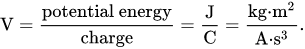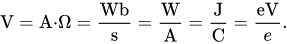# Volt (V)

### Volt definition

One volt is defined as the electric potential between two points of a conducting wire when an electric current of one ampere dissipates one watt of power between those points. Equivalently, it is the potential difference between two points that will impart one joule of energy per coulomb of charge that passes through it. It can be expressed in terms of SI base units (m, kg, s, and A) as:It can also be expressed as amperes times ohms (current times resistance, Ohm's law), webers per second (magnetic flux per time), watts per ampere (power per unit current, definition of electric power), or joules per coulomb (energy per unit charge), which is also equivalent to electronvolts per elementary charge:One Volt is defined as energy consumption of one joule per electric charge of one coulomb.

1V = 1J/C

One volt is equal to current of 1 amp times resistance of 1 ohm:

1V = 1A ⋅ 1Ω

### Alessandro Volta

The Volt unit is named after Alessandro Volta, an Italian physicist who invented an electric battery.

### Volt subunits and conversion table

name symbol conversion example
microvolt μV 1μV = 10-6V V = 30μV
millivolt mV 1mV = 10-3V V = 5mV
volt V

-

V = 10V
kilovolt kV 1kV = 103V V = 2kV
megavolt MV 1MV = 106V V = 5MV

### Volts to watts conversion

The power in watts (W) is equal to the voltage in volts (V) times the current in amps (A):

watts (W) = volts (V) × amps (A)

### Volts to joules conversion

The energy in joules (J) is equal to the voltage in volts (V) times the electric charge in coulombs (C):

joules (J) = volts (V) × coulombs (C)

### Volts to amps conversion

The current in amps (A) is equal to the voltage in volts (V) divided by the resistance in ohms (Ω):

amps (A) = volts (V) / ohms(Ω)

The current in amps (A) is equal to the power in watts (W) divided by the voltage in volts (V):

amps (A) = watts (W) / volts (V)

### Volts to electron-volts conversion

The energy in electronvolts (eV) is equal to the potential difference or voltage in volts (V) times the electric charge in electron charges (e):

electronvolts (eV) = volts (V) × electron-charge (e)

= volts (V) × 1.602176e-19 coulombs (C)

### Common voltages

The voltage produced by each electrochemical cell in a battery is determined by the chemistry of that cell (see Galvanic cell § Cell voltage). Cells can be combined in series for multiples of that voltage, or additional circuitry added to adjust the voltage to a different level. Mechanical generators can usually be constructed to any voltage in a range of feasibility.

Nominal voltages of familiar sources:

• Nerve cell resting potential: ~75 mV
• Single-cell, rechargeable NiMH or NiCd battery: 1.2 V
• Single-cell, non-rechargeable (e.g., AAA, AA, C and D cells): alkaline battery: 1.5 V; zinc-carbon battery: 1.56 V if fresh and unused
• LiFePO4 rechargeable battery: 3.3 V
• Cobalt-based Lithium polymer rechargeable battery: 3.75 V (see Comparison of commercial battery types)
• Transistor-transistor logic/CMOS (TTL) power supply: 5 V
• USB: 5 V DC
• PP3 battery: 9 V
• Automobile battery systems are 2.1 volts per cell; a "12V" battery is 6 cells or 12.6V; a "24V" battery is 12 cells or 25.2V. Some antique vehicles use "6V" 3-cell batteries or 6.3 volts.
• Household mains electricity AC: (see List of countries with mains power plugs, voltages and frequencies)
• 100 V in Japan
• 120 V in North America,
• 230 V in Europe, Asia, Africa and Australia
• Rapid transit third rail: 600–750 V (see List of railway electrification systems)
• High-speed train overhead power lines: 25 kV at 50 Hz, but see the List of railway electrification systems and 25 kV at 60 Hz for exceptions.
• High-voltage electric power transmission lines: 110 kV and up (1.15 MV is the record; the highest active voltage is 1.10 MV)
• Lightning:, often around 100 MV.
Source: Wikipedia

Currently, we have around 5661 calculators, conversion tables and usefull online tools and software features for students, teaching and teachers, designers and simply for everyone.